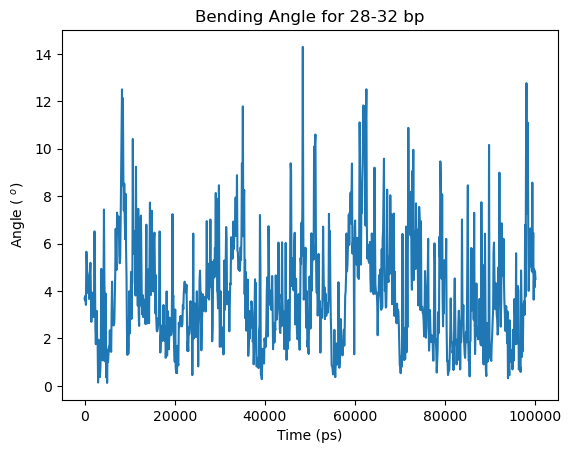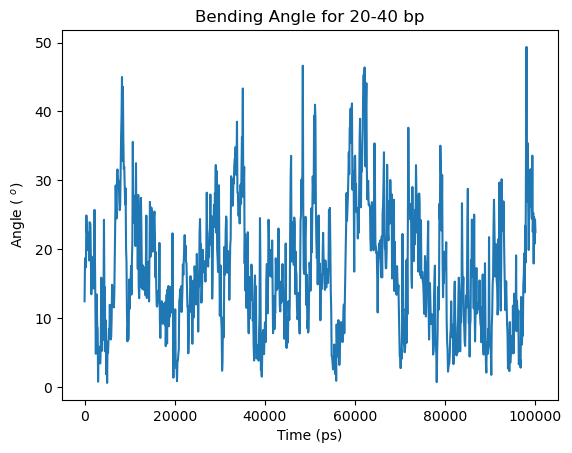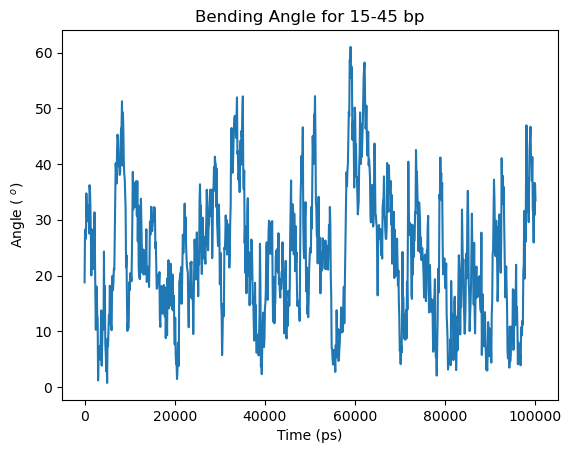# Analysis of Helical Axis¶

• This tutorial discuss the analyses that can be performed using the dnaMD Python module included in the do_x3dna package. The tutorial is prepared using Jupyter Notebook and this notebook tutorial file could be downloaded from this link.
• HelAxis_odna.dat input file is required in this tutorial. This file is generated from do_x3dna using the trajectory, which contains the free DNA. These two file should be present inside tutorial_data of the current/present working directory.
• The Python APIs should be only used when do_x3dna is executed with -ref option.
• Detailed documentation is provided here.

## Importing Python Modules¶

• numpy: Required for the calculations involving large arrays
• matplotlib: Required to plot the results
• dnaMD: Python module to analyze DNA/RNA structures from the do_x3dna output files.
In :

import numpy as np
import matplotlib.pyplot as plt
import dnaMD
%matplotlib inline


## Initializing DNA object and storing data to it¶

• DNA object is initialized by using the total number of base-pairs
• Local helical axis is formed by two adjacent base-pairs. Therefore, total number of Helical-axis postion is less than one of total number of base-pairs.
• X, Y and Z positions (Helical X-axis, Helical Y-axis and Helical Z-axis) of the Helical axis can be read and stored in DNA object from the input file using function set_helical_axis(…).
• To speed up processing and analysis, data can be stored in a HDF5 file by including HDF5 file name as a argument during initialization. Same file can be used to store and retrieve all other parameters.
In :

## Initialization
fdna = dnaMD.DNA(60)     #Initialization for 60 base-pairs free DNA

## If HDF5 file is used to store/save data use these:
# fdna = dnaMD.DNA(60, filename='odna.h5')     #Initialization for 60 base-pairs free DNA

# Number of local helical axis positions = Number of base-pairs - one
# Number of helcial steps in a 60 base-pairs DNA = 59
fdna.set_helical_axis('tutorial_data/HelAxis_odna.dat')




## Smoothening of Helical Axis¶

• The helical axis postions calculated from do_x3dna are localized and do not form a smooth global axis upon joining these positions.
• The helical axis could be smoothed by spline interpolation using method generate_smooth_axis(…).
• A smooth three dimensional curve is fitted along the positions of local helical axis by spline interpolation method implemented in SciPy package.
• This procedure might take long time on large trajectory due to the fitting procedure.

Warning: Lower value of smooth may lead to an artifact of local sharp kink in the smoothed axis. Higher value of smooth may lead to the calculation of wrong helical axis.

In :

### Smoothening of helical axis
fdna.generate_smooth_axis(smooth=500, spline=3, fill_point=6)

Fitting spline curve on helcial axis of frame 1000 out of 1001 frames
Finished spline curve fitting...


## Extraction of original and smoothed helical axis postions as a function of time (manually)¶

• Psotions of original and smoothed helical axis for the given base-steps range can be extracted from the DNA obejct using function dnaMD.DNA.get_parameters(…).
• Following example shows, how to extract the data.
In :

### Extraction of original helical axis for 5-55 base-steps segment

# X-axis original
RawX, bp_idx = fdna.get_parameters('helical x-axis', [5, 55], bp_range=True)
# Y-axis original
RawY, bp_idx = fdna.get_parameters('helical y-axis', [5, 55], bp_range=True)
# Z-axis original
RawZ, bp_idx = fdna.get_parameters('helical z-axis', [5, 55], bp_range=True)

# X-axis smoothed
SmoothX, bp_idx = fdna.get_parameters('helical x-axis smooth', [5, 55], bp_range=True)
# Y-axis smoothed
SmoothY, bp_idx = fdna.get_parameters('helical y-axis smooth', [5, 55], bp_range=True)
# Z-axis smoothed
SmoothZ, bp_idx = fdna.get_parameters('helical z-axis smooth', [5, 55], bp_range=True)

# Here RawX is a 2D array of shape (base-step, nframes)

# Some examples
## x, y, z coordinates of nth base-step in mth frame: base-step index = (n - 5), frame index = (m - 1)
print ( "\n====== Some Examples ======" )
print ("Original coordinates of 8th base-step in 15th frame  : [ %8.3f %8.3f %8.3f ]" %
(RawX, RawY, RawZ))
print ("Smoothened coordinates of 8th base-step in 15th frame: [ %8.3f %8.3f %8.3f ]" %
(SmoothX, SmoothY, SmoothZ))

print ("\nOriginal coordinates of 40th base-step in 900th frame  : [ %8.3f %8.3f %8.3f ]" %
(RawX, RawY, RawZ))
print ("Smoothened coordinates of 40th base-step in 900th frame: [ %8.3f %8.3f %8.3f ]\n" %
(SmoothX, SmoothY, SmoothZ))


====== Some Examples ======
Original coordinates of 8th base-step in 15th frame  : [  101.280  173.630   84.670 ]
Smoothened coordinates of 8th base-step in 15th frame: [  106.443  172.187   85.432 ]

Original coordinates of 40th base-step in 900th frame  : [  208.140  171.130   79.730 ]
Smoothened coordinates of 40th base-step in 900th frame: [  205.639  172.066   80.286 ]



## To calculate curvature and tangent vectors along helical axis¶

• The bending at a specifc position on the helical axis can be quantifed by its curvature.
• Approximate bending of a DNA segment could be also quantified by the angle between the tangent vectors of the segment’s end points
• Both curvature and tangent vectors could be calculated using dnaMD.DNA.calculate_curvature_tangent(…) function.
• Curvature and tangent vectors should be calculated after generating the smooth helical axis.
In :

### Calculating curvature and tangent vectors
# If store_tangent=True; then tangent vectors will be stored for later use, otherwise it will be discarded
fdna.calculate_curvature_tangent(store_tangent=True)
fdna.calculate_angle_bw_tangents([5,50])

# Curvature vs Time for 22nd bp
plt.title('Curvature for 22nd bp')
time, value = fdna.time_vs_parameter('curvature', )
plt.plot(time, value)
plt.xlabel('Time (ps)')
plt.ylabel('Curvature ($\AA^{-1}$)')
plt.show()

# Total Curvature vs Time for 10-50 bp segment
plt.title('Total Curvature for 10-50 bp segment')
# Bound DNA
# Here, Total Curvature is considered as the sum over the local curvatures of the base-steps
time, value = fdna.time_vs_parameter('curvature', [10, 50], merge=True, merge_method='sum')
plt.plot(time, value)

plt.xlabel('Time (ps)')
plt.ylabel('Curvature ($\AA^{-1}$)')
plt.show()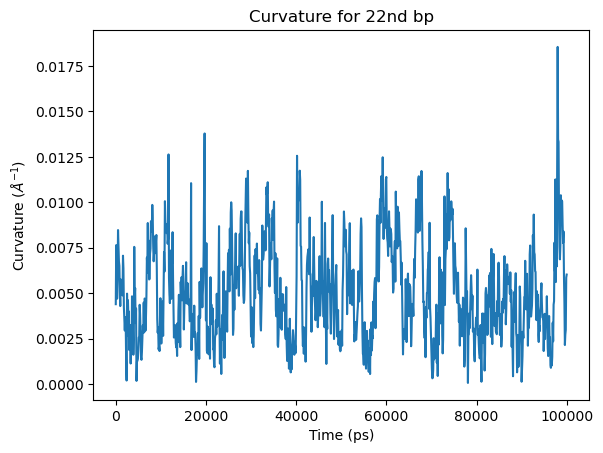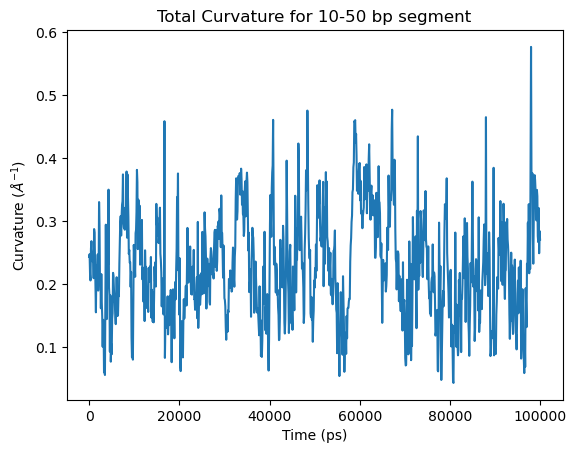## Writing trajectory of Helical Axis¶

• Calculated helical axis from do_x3dna and smoothed helical axis could be written as trajectory in PDB format file using dnaMD.DNA.write_haxis_pdb(…)
• If write_smooth_axis=True: Coordinates of smoothed helical axis will be written
• If write_orig_axis=True: Coordinates of original helical axis will be written
• If write_curv=True: The calculated curvature of smooth helical axis will be written in B-factor field of PDB file. This might be helpful to visualize the helical axis according to its bending in color scale.
• The values of curvature might be very small ( < 0.01 ). Therefore, scaling up of curvature by 100 to 1000 times may help in better visualization on color scale.
In :

# Only smoothed helical axis
fdna.write_haxis_pdb(filename='only_smoothed_axis.pdb', write_smooth_axis=True)

# Only original helical axis
fdna.write_haxis_pdb(filename='only_original_axis.pdb', write_smooth_axis=False, write_orig_axis=True)

# Both original and smoothed axis
fdna.write_haxis_pdb(filename='original_smoothed_axis.pdb', write_smooth_axis=True, write_orig_axis=True)

# Both original and smoothed axis with curvature scaled-up by 1000 times
fdna.write_haxis_pdb(filename='original_smoothed_axis_curvature_.pdb', write_smooth_axis=True, write_orig_axis=True,
write_curv=True, scale_curv=1000)


## Angle between tangent vectors¶

• Approximate bending of a DNA segment could be also quantified by the angle between the tangent vectors of the segment’s end points
• Angle between the tangent vectors can be calculated using dnaMD.DNA.calculate_angle_bw_tangents(…)
In :

# Angle vs Time for 28-32 bp
plt.title('Bending Angle for 28-32 bp')

# Calculating angle between the tangent vectors of 38th and 32nd base-steps
angle = fdna.calculate_angle_bw_tangents([28,32])
# Change to Degree
angle = np.degrees(angle)
# Plotting
plt.plot(fdna.time, angle)
plt.xlabel('Time (ps)')
plt.ylabel('Angle ( $^o$)')
plt.show()

# Angle vs Time for 25-35 bp
plt.title('Bending Angle for 25-35 bp')

# Calculating angle between the tangent vectors of 25th and 35th base-steps
angle = fdna.calculate_angle_bw_tangents([25,35])
# Change to Degree
angle = np.degrees(angle)
# Plotting
plt.plot(fdna.time, angle)
plt.xlabel('Time (ps)')
plt.ylabel('Angle ( $^o$)')
plt.show()

# Angle vs Time for 20-40 bp
plt.title('Bending Angle for 20-40 bp')

# Calculating angle between the tangent vectors of 20th and 40th base-steps
angle = fdna.calculate_angle_bw_tangents([20,40])
# Change to Degree
angle = np.degrees(angle)
# Plotting
plt.plot(fdna.time, angle)
plt.xlabel('Time (ps)')
plt.ylabel('Angle ( $^o$)')
plt.show()

# Angle vs Time for 15-45 bp
plt.title('Bending Angle for 15-45 bp')

# Calculating angle between the tangent vectors of 15th and 45th base-steps
angle = fdna.calculate_angle_bw_tangents([15,45])
# Change to Degree
angle = np.degrees(angle)
# Plotting
plt.plot(fdna.time, angle)
plt.xlabel('Time (ps)')
plt.ylabel('Angle ( $^o$)')
plt.show()

# Angle vs Time for 5-55 bp
plt.title('Bending Angle for 5-55 bp')

# Calculating angle between the tangent vectors of 5th and 55th base-steps
angle = fdna.calculate_angle_bw_tangents([5,55])
# Change to Degree
angle = np.degrees(angle)
# Plotting
plt.plot(fdna.time, angle)
plt.xlabel('Time (ps)')
plt.ylabel('Angle ( $^o$)')
plt.show()